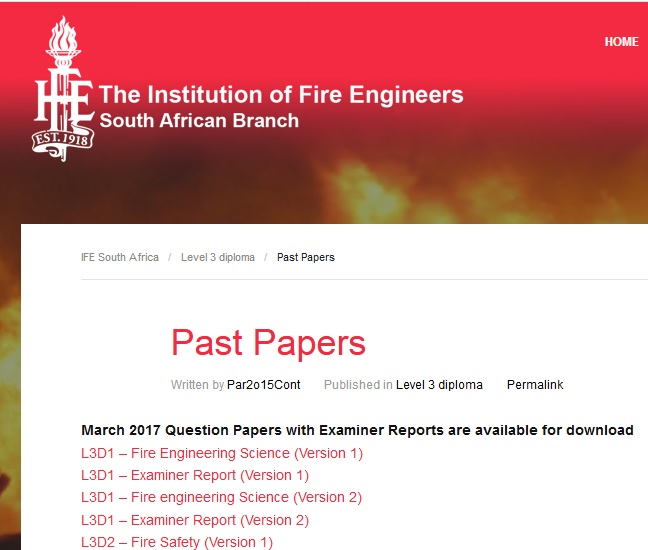1. Home
2. »
3. Question Paper
4. »
5. IFE Level 4 Certificate Examination : Institu...
All posts from

# IFE Level 4 Certificate Examination : Institution of Fire Engineers

Organisation : IFE – The Institution of Fire Engineers
Exam : IFE – Level 4 Certificate Examination
Year: 2016
Document Type : Sample Question Paper
Category or Subject : All Subject

Want to comment on this post?

Website : http://www.ife.org.za/past-papers/#
Civil Emergency and Disaster Management : https://www.southafricain.com/uploads/7108-L4Ccivil.pdf

## IFE – Level 4 Certificate Examination

Fire Engineering Science :

### Question 1

a) Using the energy equation and an annotated diagram, explain the operating principle of a Pitot Tube. (10 marks)

Related : IFE Level 4 Certificate Examination Syllabus : www.southafricain.com/7090.html

b) A Pitot Tube fitted to the nose of an aircraft flying at an altitude of 4000m indicates a static pressure of 6.6×104 Pa. Calculate the velocity of the aircraft. Take the density of air at 4000m to be 0.8kgm-3 and the pressure of the air at 4000m as 6.2×104 Pa. (10 marks)

### Question 2

A cylindrical steel tank 300cm in diameter and 600cm in height stands on a lattice support such that its base is 125cm from the ground. The tank is completely filled with Sodium Hydroxide solution. The tank contents are leaking from a 15cm diameter hole, the centre of which is 200cm from the top of the tank. (Density of NaOH solution is given as 1.4g/ml)

a) Calculate, to three decimal places, the mass of liquid in the tank before any leakage. Show all calculations. (3 marks)
b) Calculate, to three decimal places, the velocity of the liquid as it begins to escape the tank. Show all calculations. (10 marks)

c) What would be the velocity of the liquid if the hole were 100cm in diameter? Give your answer to three decimal places. Compare this value with that in b) and briefly explain the difference. Show all calculations. (5 marks)

d) How would the velocity change if the tank was positioned on the floor instead of on a stand 125cm off the ground? Briefly explain your answer. (2 marks)### Question 3

a) Describe five factors that affect the rate of combustion of timber. (5 marks)
b) What does activation energy mean? How does it affect the flammability of a substance? (3 marks)

c) Describe how free radicals are formed in combustion with particular reference to homolytic cleaving and show how their production is part of a chain reaction. (6 marks)
d) Chain reactions involving free radicals can usually be divided into three distinct processes. Describe each of the three processes. (6 marks)

### Question 4

a) Write a balanced equation that describes the combustion of ethane in air. (5 marks)
b) Hc is the term given for the heat of combustion for a substance. For ethane, these values are Explain why two different values are given. (4 marks)
c) The values for ??Hc (ethane) are arrived at using a Bomb Calorimeter. Give three reasons why the results obtained from such a device are reliable and repeatable. (3 marks)

d) Use your balanced equation from part a) and the data below to calculate a value for ??Hf for oxygen. Explain the value arrived at.
Hf (ethane) = -83.7 KJMol-1
Hf (carbon dioxide) = -392.6 KJMol-1
Hf (water) = -240.5 KJMol-1 (vapour) (8 marks)

### Question 5

a) The terms used to classify chemicals for international transportation have recently been standardised to the UN Globally Harmonized (UNGH) System. Under this system, explain how flammable liquids are defined and how they are divided into categories. (8 marks)

b) Published standards and guides give recommendations and guidance for the control of fire and explosion risks on sites at which chemicals are stored or processed in significant quantities. When selecting a site on which to erect a petro-chemical installation, describe the factors that should be considered in order to control the risk of fire and explosion. (12 marks)

Question 6 :
Consider the electrical circuit below. (Note that this circuit has two loops that are connected at junctions X and Y and each loop contains a cell and resistor/s.)
a) Identify and state the rule that governs the value of current flowing in and out of junctions within an electric circuit. (3 marks)

b) Identify and state the law that governs the changes in potential difference around a loop circuit such as above. (3 marks)
c) Use these two rules to calculate the value of the current at each of the points A, B and C. Show all calculations. (14 marks)

Question 7 :
a) Explain the following terms that relate to protective devices in electric circuits.
i. Operating Current
ii. Fusing Factor
iii. Breaking Capacity
iv. Short Circuit Current (8 marks)
b) Compare the semi-enclosed re-wireable fuse with the H.B.C (high breaking capacity) fuse. Your answer should include advantages and disadvantages for each device. (12 marks)

Question 8 :
a) Orientation is one factor that affects the rate of spread of flame in solids. Identify and explain six additional factors. (18 marks)

b) Consider the two situations in the diagram below. They each show the free burning of identical materials in identical rooms. Situation 1 shows burning against a non- combustible wall; situation 2 shows the fire is in the centre of the room. There is no limit to the amount of oxygen available for combustion. Compare the temperatures t1 and t2 of the flame taken at the same height h and at the same time in the fire’s development in each case. Explain your answer. (2 marks)